# Unclear step in "Change of variable in a multiple integral" proof

Unconscious
I'm studying the proof of this theorem (Zorich, Mathematical Analysis II, 1st ed., pag.136):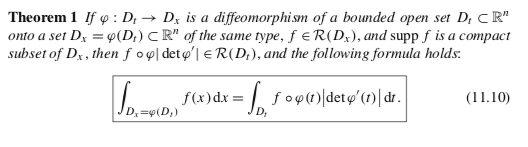which as the main idea uses the fact that a diffeomorphism between two open sets can always be locally decomposed in a composition of elementary ones.
As a remark, an elementary diffeomorphism ##\varphi## is a diffeomorphism under which only a single variable is modified (in the following example, the last one):

##\left\{\begin{matrix}
x^1=\varphi^1(t^1,...,t^n)=t^1\\
x^2=\varphi^2(t^1,...,t^n)=t^2\\
...\\
x^{n-1}=\varphi^{n-1}(t^1,...,t^n)=t^{n-1}\\
x^{n}=\varphi^{n}(t^1,...,t^n)
\end{matrix}\right.##

The proposed proof (pag.142) starts in this way: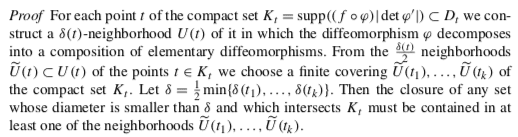I can't understand the last sentence, because as an intuitively counterexample I'm imagining out this situation: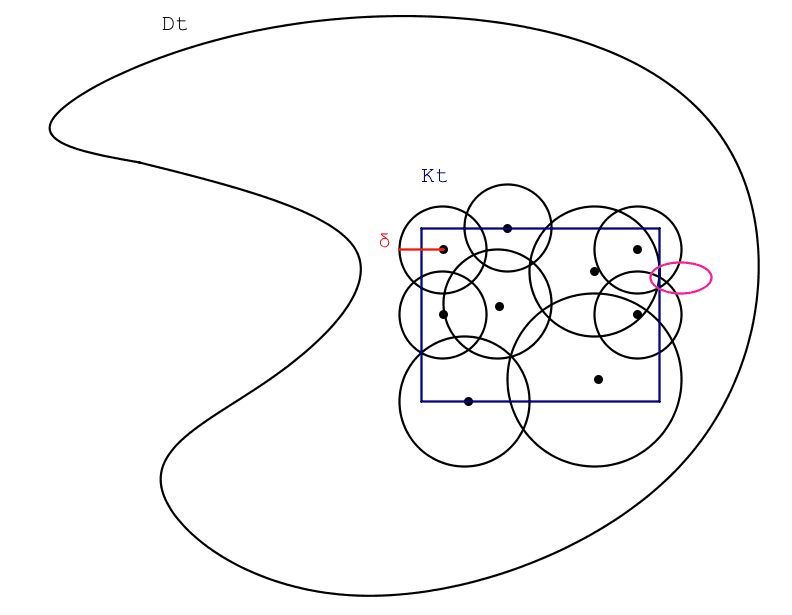where the pink set is a set with a diameter less than ##\delta##, that intersect ##K_t##, but that is not contained in any neighborhoods of the finite family covering ##K_t##.
Am I missing something?

Homework Helper
2022 Award
I think $U(t_1), \dots, U(t_k)$ is meant. The truth of that is a consequence of the triangle rule, and the consequence is that any sufficiently small neighbourhood is contained within a neighbourhood where $\varphi$ decomposes as claimed. (Compare the proof of the Heine-Cantor Theorem.)

Unconscious
Ok, the idea is clear. Thank you.

Unconscious
I post again in this thread, because I have another question on the same argument.
I'm studying this generalization (pag.145) of the previous theorem: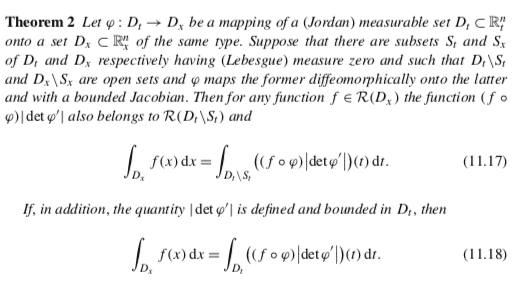whose proof begins in this way: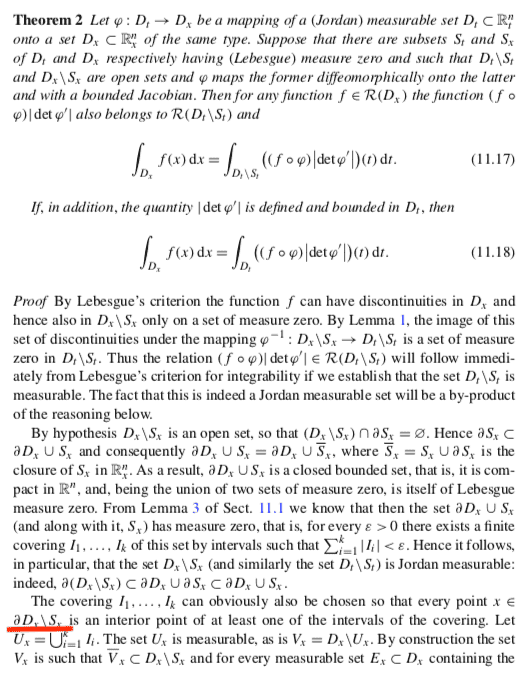where my doubt is on the highlighted relation.
I think that it would be ##x\in\partial D_x \cup S_x## instead ##x\in\partial D_x \setminus S_x##, no?
If I'm wrong, then I can't understand the successive relation ##\overline{V}_x\subset D_x\setminus S_x##, because I can't see why a point of ##\partial V_x## could not be inside ##S_x##.

Last edited: